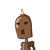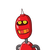# [tex]koi \: bhi \: noi \: h \: kya[/tex]= 182 x 22/7 = 26 x 22 = 572 cm?– A toy is in the form of a

[tex]koi \: bhi \: noi \: h \: kya[/tex]

= 182 x 22/7 = 26 x 22 = 572 cm?
– A toy is in the form of a cone of radius 3.5 cm
mounted on a hemisphere of same radius. The
total height of the toy is 15.5 cm. Find the total
surface area of the toy.​​

### 2 thoughts on “<br />[tex]koi \: bhi \: noi \: h \: kya[/tex]<br /><br /><br />= 182 x 22/7 = 26 x 22 = 572 cm?<br />– A toy is in the form of a”

1.214.5 cm²

Step-by-step explanation:

Given:

➜ A toy is in the form of a cone of radius 3.5 cm mounted on a hemisphere of same radius. The total height of the toy is 15.5 cm.

Find:

➜ Find the total surface area of the toy.

According to the given question:

➜ 3.5 cm = radius of the cone and the hemisphere.

➜ 15.5 cm = total height of the toy.

➜ 12 cm = height of the cone = (3.5 – 15.5 = 12cm).

Calculations:

➜ Slant height of cone (I) = √h² + r²

➜ I = √12² + (3.5)²

➜ I = √12² + (7/2)²

➜ I = √144 + 49/4 = √(576 + 49)/4 = √625/4

➜ I = 25/2

Formulas:

πrl is curved surface area of cone.

2πr2 is the curved surface area of the hemisphere.

➜ (22/7 × 7/2 × 25/2) = 275/2 cm²

➜ 2 × 22/7 × (7/2)

➜ 2 77 cm²

Formula:

Total surface area of toy = Curved Surface Area of cone + Curved Surface Area of hemisphere.

➜ (275/2 + 77) cm²

➜ (275 + 154)/2 cm²

➜ 429/2 cm²

➜ 214.5 cm²

Therefore, 214.5 cm² is the total surface area of the toy.

2.214.5cm²

Step-by-step explanation:

Total surface area of toy = Curved surface area of cone + surface area of hemisphere

Curved surface area of cone = πrl

=> Where r = 3.5cm,

=> Height = 15.5 − 3.5 = 12cm

And hence, l=12.5cm(by using formula l²=h²+b²)

Therefore C.S.A. of cone = π×3.5×12.5

= 137.5cm²

Surface area of hemisphere =2πr²

=2×π×(3.5)²

=77cm²

Hence T.S.A of toy = 77+137.5

= 214.5cm²